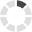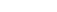### Site InformationLoading... Please wait...

# SEC 280 Final Exam Answers (DeVry)

\$30.00

## Product Description

SEC 280 SEC280 Final Exam Answers (DeVry)

 1. (TCO 5) Unsolicited commercial e-mail is known as _____. (Points : 4)
 Question 2.2. (TCO 5) Two tools that can be used to encrypt e-mail are _____. (Points : 4)
 Question 3.3. (TCO 5) One of the largest security problems with IM programs is _____. (Points : 4)
 Question 4.4. (TCO 6) What is the name of the process that controls access to information based on the sensitivity of that information and whether or not the user is operating at the appropriate sensitivity level and has the authority to access that information? (Points : 4)
 Question 5.5. (TCO 6) A network administrator wants to be sure that when users change their passwords, they do not reuse a previous password. What domain password policy will need to be configured? (Points : 4)
 Question 6.6. (TCO 6) A network administrator wants to specify the number of days a password must be used before it can be changed again. What domain password policy will need to be configured? (Points : 4)
 Question 7.7. (TCO 6) Which of the following can a user control on their system? (Points : 4)
 Question 8.8. (TCO 6) Which is a 100% secure method to download applications from the Internet? (Points : 4)
 Question 9.9. (TCO 6) SSMTP uses TCP port (Points : 4)
 Question 10.10. (TCO 2) HTTPS uses TCP port (Points : 4)
 Question 11.11. (TCO 2) When an attacker attempts to get credit card numbers using telephone and voice technologies, it's called (Points : 4)
 Question 12.12. (TCO 8) Which is the law that overhauled the financial accounting standards for publicly traded firms in the United States? (Points : 4)
 Question 13.13. (TCO 4) Which of the following is not one of the three forms of trust models? (Points : 4)
 Question 14.14. (TCO 4) Once you have properly obtained a digital certificate for a website (Points : 4)
 Question 15.15. (TCO 2) For products evaluated by Common Criteria, the highest level of assurance is (Points : 4)
 Question 16.16. (TCO 2) HTTPS uses port (Points : 4)
 Question 17.17. (TCO 3) Which transport layer protocol is connection-oriented? (Points : 4)
 Question 18.18. (TCO 3) Packets delivered to a network, such as an office LAN, are usually sent using the destination system's (Points : 4)
 Question 19.19. (TCO 3) Packet delivery to distant systems is usually accomplished by the use of (Points : 4)
 Question 20.20. (TCO 3) The series of worldwide interconnected networks is referred to as the (Points : 4)
 Question 21.21. (TCO 3) The network that is an extension of a selected portion of a company's intranet to external partners is referred to as the (Points : 4)
 Question 22.22. (TCO 6) You are building a network and need to connect five computers in an office. They will be on the same network segment and you should be able to perform some security functions, such as port address security. Which device would you use to connect the computers? (Points : 4)
 Question 23.23. (TCO 6) The following are steps in securing a workstation EXCEPT which one? (Points : 4)
 Question 24.24. (TCO 6) Which device converts analog signals to digital signals and vice versa? (Points : 4)
 Question 25.25. (TCO 6) Centralized monitoring of the health of the network is the function of a (Points : 4)

Question 26.26. (TCO 2) Give an example of a hoax and how it might actually be destructive. (Points : 10)

Question 27.27. (TCO 3) Briefly describe a public key infrastructure (PKI). (Points : 10)

Question 28.28. (TCO 3) How does PGP work? (Points : 10)

Question 29.29. (TCO 3) What is the difference between TCP and UDP? (Points : 10)

Question 30.30. (TCO 4) What are the laws that govern computer access and trespass? (Points : 10)

Question 31.31. (TCO 5) What are the three steps of establishing proper privileges? (Points : 10)

Question 32.32. (TCO 5) Define spam. (Points : 10)

Question 33.33. (TCO 5) List three approaches that antispam software uses to filter out junk e-mail. (Points : 10)

Question 34.34. (TCO 6) What are the four common methods for connecting equipment at the physical layer? (Points : 10)

Question 35.35. (TCO 6) What are the differences among user, group, and role management? (Points : 10)

Question 36.36. (TCO 8) What are the laws that govern computer access and trespass? (Points : 10)

Question 37.37. (TCO 8) What is PII as it relates to privacy? (Points : 10)

Question 38.38. (TCO 9) Describe methods of account management (SSO, time of day, logical token, and account expiration). (Points : 10)

Question 39.39. (TCO 9) What is the importance of a baseline to change management? (Points : 10)

Question 40.40. (TCO 6) What are the concepts of the capability maturity model integration? (Points : 10)

Question 41.41. (TCOs 1, 2, and 3) In an e-commerce agreement between two parties, why is non-repudiation important? (Points : 10)

## Customers also viewed

• \$22.99• \$30.00• \$35.00• \$6.99• \$30.00## Related Products

• SEC 280 SEC280 Week 8 Final Exam \$35.00• SEC 280 Final Exam with Answers \$22.99• SEC 360 Final Exam with Answers \$24.99• DeVry HIM 355 Midterm Exam Answers \$24.99• Devry SCI 228 Final Exam Answers \$29.99Click the button below to add the SEC 280 Final Exam Answers (DeVry) to your wish list.